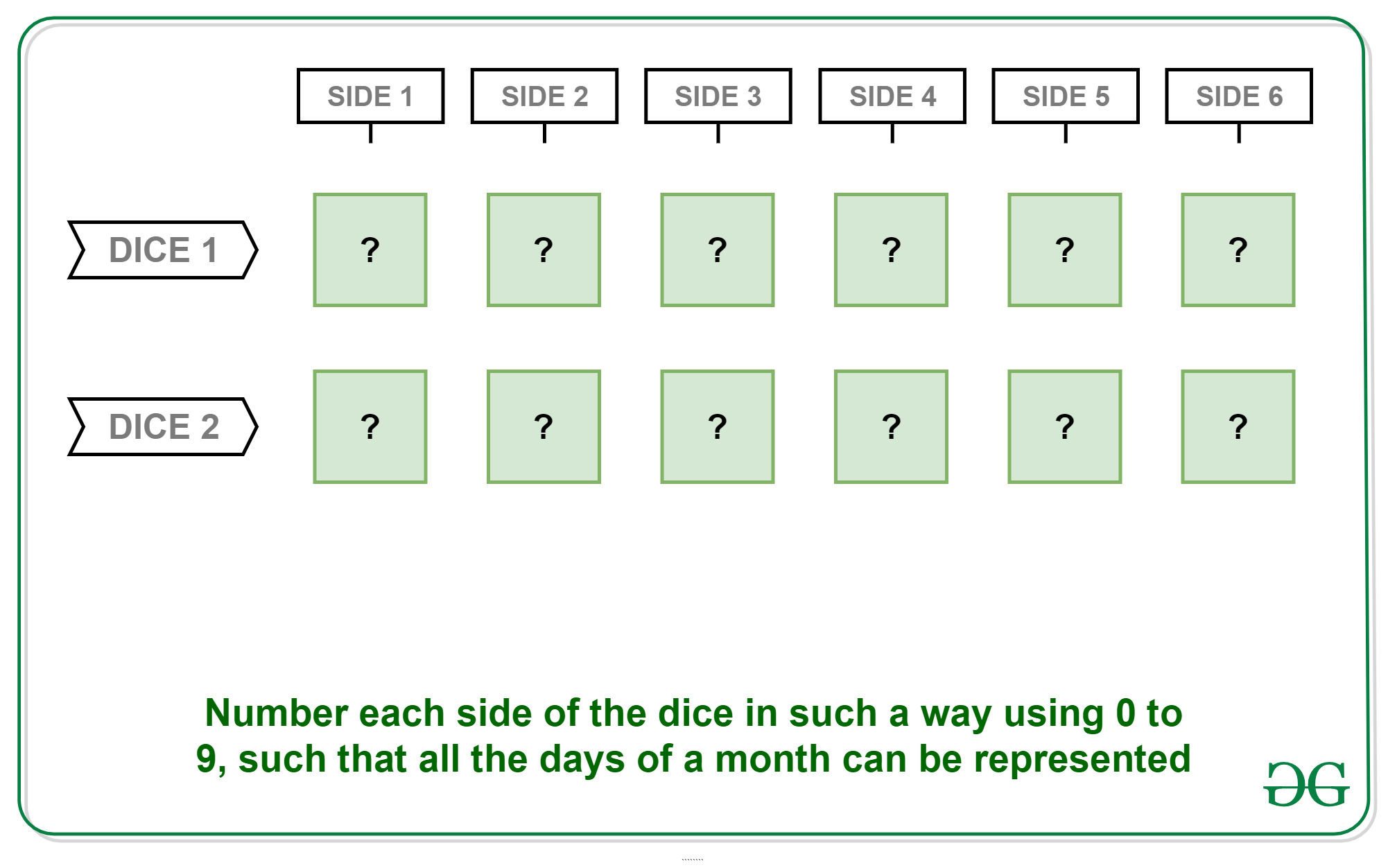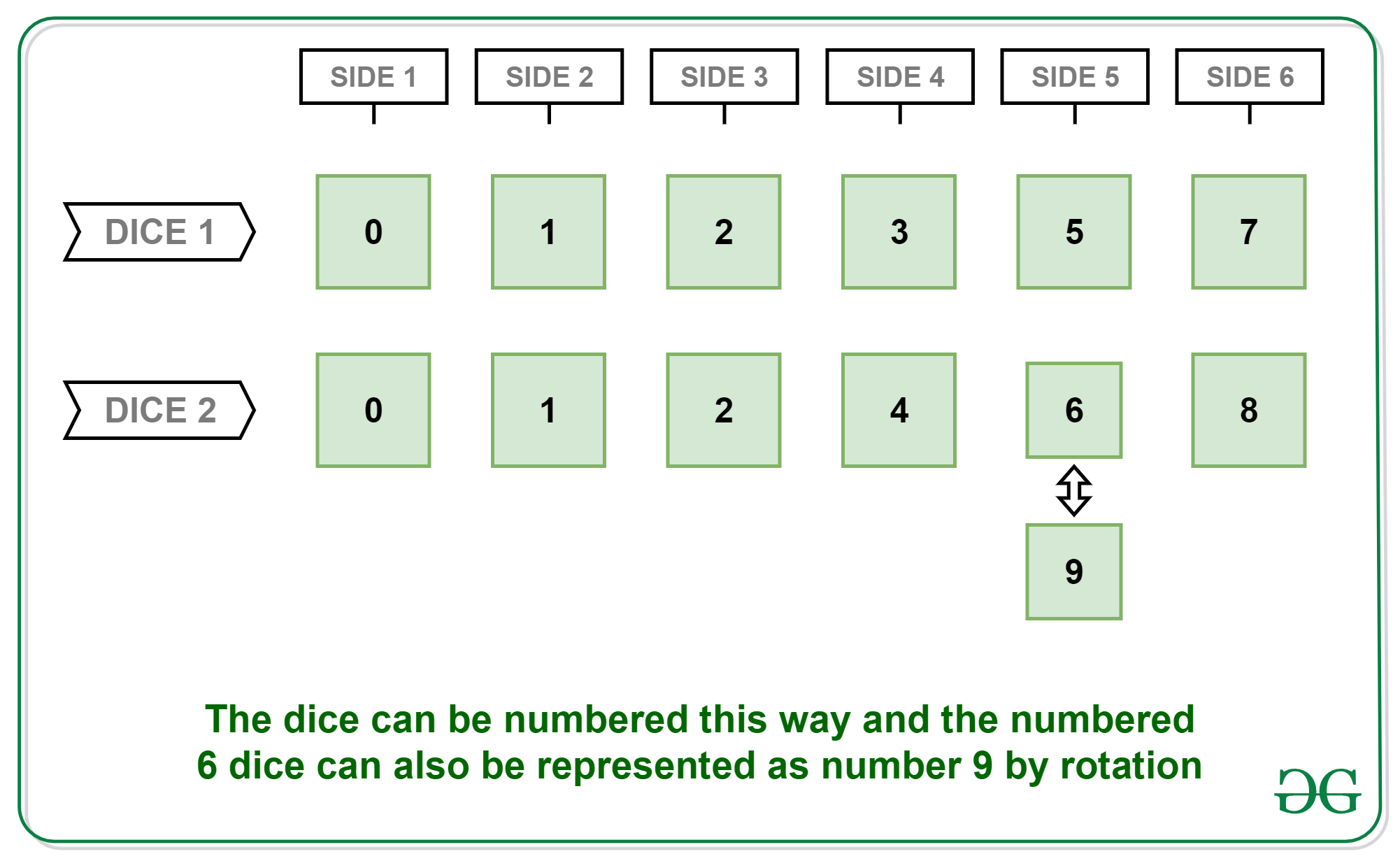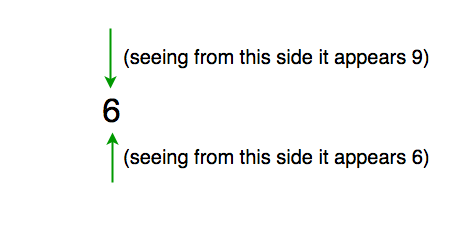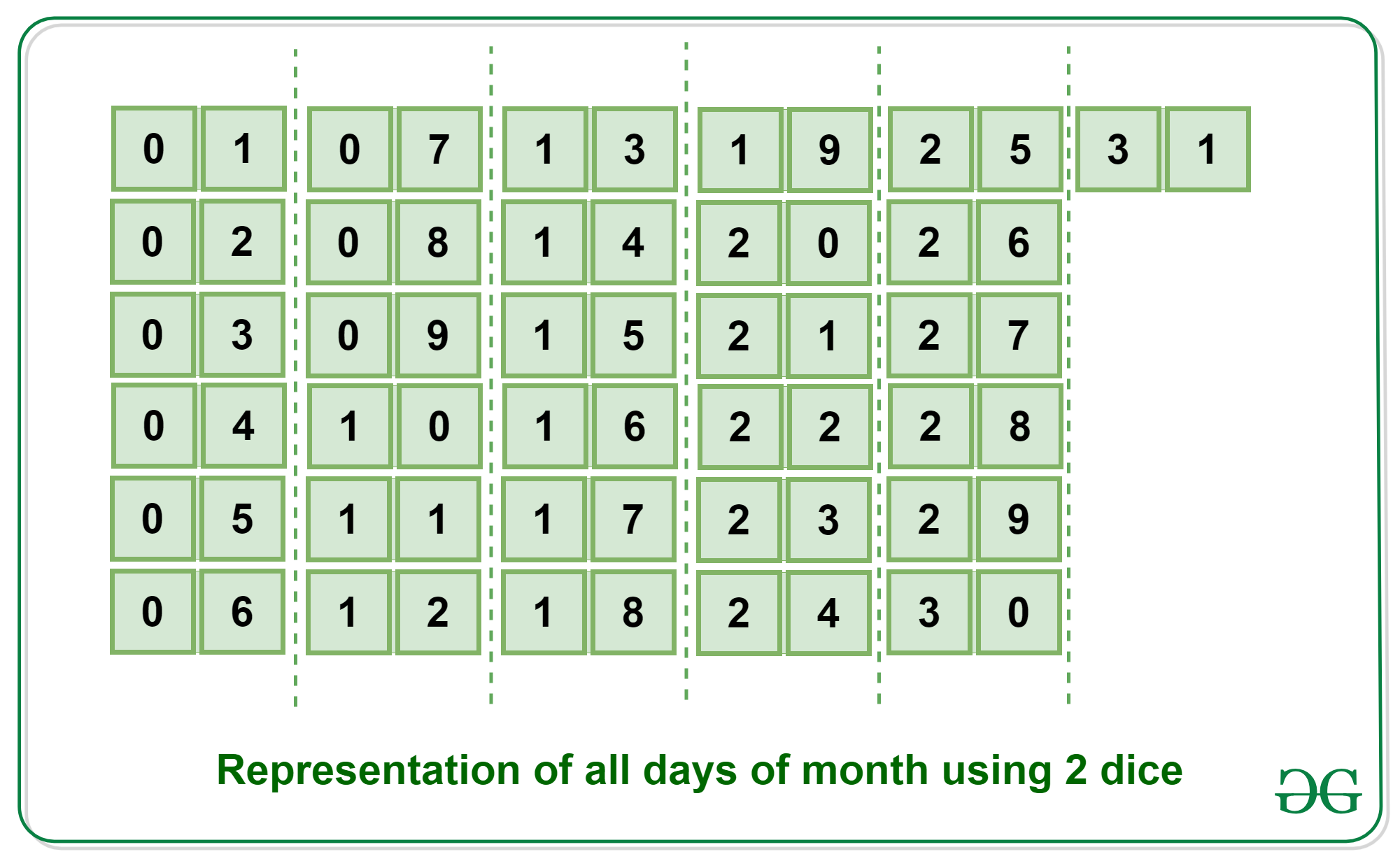GeeksforGeeks App
Open AppBrowser
Continue

# Puzzle 23 | (Days of month using 2 dice)

Puzzle: How can you represent days of month using two 6 sided dice? You can write one number on each face of the dice from 0 to 9 and you have to represent days from 1 to 31, for example for 1, one dice should show 0 and another should show 1, similarly for 29 one dice should show 2 and another should show 9.Dice 1: 0 1 2 3 5 7
Dice 2: 0 1 2 4 6 8

Explanation:You have to show 11, 22 so 1 and 2 should be present in both dices, similarly to show 01, 09. 0 should be present in both dices, now the trick is for showing 9 you can use dice with 6 printed on one of the faces.Here is the overall representation of all the days in 2 dice:Detail Explanation:

Since the last digit varies from 0 to 9 and the first digit from 0 to 3, which means 0, 1, 2 must be there in each dice. 3 is not needed in both dice because in a month only 30 and 31st dates exist, so they can be made because we will have 1 and 0 in each dice.

Now we have total sides = 6+6=12.

Total numbers we want to have on dice are = 10 (0 to 9) + 3 (0 to 2) = 13.

Here we have one extra number to represent.

So to compensate that no we use a trick here that when we rotate 6 it becomes 9, so we don’t need to write 9 on the dice.

So now total sides = 12 and total numbers to write are also 12.

The important thing here is 0,1,2 must be there on both the dice, the rest we may put on any dice as per our convenience.

So few solutions can be –

Sol1:

Dice1: 0, 1, 2, 3, 4, 5.

Dice2: 0, 1, 2, 6, 7, 8.

Sol2:

Dice1: 0, 1, 2, 3, 6, 7.

Dice2: 0, 1, 2, 4, 5, 8.

Similarly, any combination can be answered just we need to take care that 0, 1, 2 must be there on both the dices.